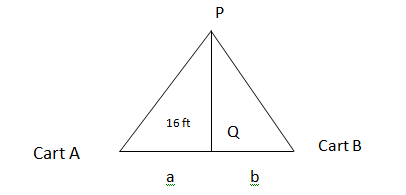# Two carts, A and B, are connected by a rope 33 ft long that passes over a pulley. The point Q is...

## Question:

Two carts, {eq}A {/eq} and {eq}B {/eq}, are connected by a rope {eq}33\ ft {/eq} long that passes over a pulley. The point {eq}Q {/eq} is on the floor {eq}16\ ft {/eq} directly beneath and between the carts. Cart {eq}A {/eq} is being pulled away from {eq}Q {/eq} at a speed {eq}5 {/eq} ft / s. How fast is cart {eq}B {/eq} moving toward {eq}Q {/eq} at the instant when cart {eq}A {/eq} is {eq}4 {/eq} ft from {eq}Q {/eq}? select the correct answer.

a. 4.94 ft / s.

b. 4.92 ft / s.

c. 5.43 ft / s.

d. 4.73 ft / s.

e. 2.73 ft / s.

## PYTHAGORAS THEOREM:

In right angle triangle we can find the sides of triangle by using Pythagoras theorem.The right angle triangle has an angle of 90{eq}^{o} {/eq} and we can find the hypotenuse by square of addition of remaining two sides. In Pythagoras theorem the base and perpendicular can be added in square form to find out the hypotenuse.

To determine the speed of cart B we have to draw a schematic diagram as:Let the length of AD is "a" and the length of BD is "b".

Here we can see in this figure that that the length of the rope APB is 33 ft and the triangle APQ and BCQ are right angles. So we can use the Pythagoras theorem to find out the length of AP and BP, we get:

{eq}\sqrt {a^2 +16^2} + \sqrt {b^2 +16^2} = 33\\ {/eq}

Now differentiating above equation with respect to time t and we get:

{eq}\dfrac{d}{dt}(\sqrt {a^2 +16^2} + \sqrt {b^2 +16^2}) = \dfrac{d}{dt}(33)\\ \dfrac{da}{dt}\left (\dfrac{a}{\sqrt {a^2 +16^2}} \right) + \dfrac{db}{dt}\left (\dfrac{b}{\sqrt {b^2 +16^2}}\right) = 0\\ {/eq}

Now we can find the length of b when a = 4 ft.

{eq}\sqrt {a^2 +16^2} + \sqrt {b^2 +16^2} = 33\\ \sqrt {4^2 +16^2} + \sqrt {b^2 +16^2} = 33\\ 16.49 + \sqrt {b^2 +16^2} =33\\ \sqrt {b^2 +16^2} = 33-16.49\\ b^2 +16^2 = 16.51^2\\ b^2 = 16.5801\\ b = \sqrt {16.5801}\\ b = 4.071 ft\\ {/eq}

now we can substitute the all values in equation (a), we get:

{eq}\dfrac{da}{dt}(\dfrac{4}{\sqrt {4^2 +16^2}} + \dfrac{db}{dt}\left (\dfrac{4.071}{\sqrt {4.071^2 +16^2}}\right ) = 0\\ {/eq}

We have :

{eq}\dfrac{da}{dt} = 5 ft/s \\ 5 * \dfrac{4}{\sqrt {4^2 +16^2}} +\dfrac{db}{dt}\left (\dfrac{4.071}{\sqrt {4.071^2 +16^2}}\right) = 0\\ 1.21 + \dfrac{db}{dt}\left (\dfrac{4.071}{\sqrt {4.071^2 +16^2}}\right ) = 0\\ \dfrac{db}{dt}\left (\dfrac{4.071}{\sqrt {4.071^2 +16^2}}\right )= -1.21\\ \dfrac{db}{dt}0.9711 =-1.21\\ \dfrac{db}{dt} = \dfrac{ -1.21}{0.9711}\\ \dfrac{db}{dt} = -2.746\\ {/eq}

Here negative sign indicates the distance is decreasing because of cart B is moving towards the direction Q with the speed approx 2.746 ft/s.